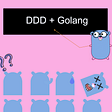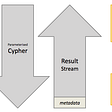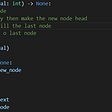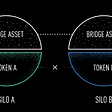# Soda Finance Hack: Could Formal Verification Have Prevented It? (Code Included)

## Where Is The Bug?!

`uint256 maximumLoan = loanInfo[_loanId].amount.mul(loanInfo[_loanId].maximumLTV).div(LTV_BASE);`
`uint256 maximumLoan = loanInfoFixed[_loanId].lockedAmount.mul(loanInfoFixed[_loanId].maximumLTV).div(LTV_BASE);`
`require(loanTotal >= maximumLoan, "collectDebt: >=");`

## Formulating The DeFi Problem

• Variable amount is an unsigned integer, so amount is integer-typed and non-negative.
• Variable lockedAmount is an unsigned integer, so lockedAmount is also integer-typed and non-negative.
• Variable loanToal is computed by function getLoanTotal() in line 106 of WETHCalculator.sol, and is actually a sum of amount and interest. Therefore, loanTotal is amount + interest and interest is also unsigned integer.
`function getLoanTotal(uint256 _loanId) public view override returns (uint256) { return getLoanPrincipal(_loanId) + getLoanInterest(_loanId); }`
• Variable maximumLoan is computed by code below,
`uint256 maximumLoan = loanInfo[_loanId].amount.mul(loanInfo[_loanId].maximumLTV).div(LTV_BASE);`
`uint256 constant LTV_BASE = 100;`
`require(_minimumLTV + 5 <= _maximumLTV, "+ 5 <= _maximumLTV"); require(_minimumLTV >= 10, ">= 10"); require(_maximumLTV <= 95, "<= 95");`
• The requirement in line 196 of WETHCalculator.sol could be modeled as loanTotal >= maximumLoan.

## Constructing The Proof in Z3 Python

`from z3 import *amount = Int('amount')interest = Int('interest')lockedAmount = Int('lockedAmount')factor = Real('factor')`
`def checkBuggy(amount, interest, factor):    s = Solver()    s.add([amount >= 0, interest >= 0])    s.add([factor > 0, factor < 1])    loanTotal = amount + interest    maximumLoan = amount * factor    s.add(loanTotal < maximumLoan)    return s.check()print(checkBuggy(amount, interest, factor))`
`def checkFixed(amount, interest, factor, lockedAmount):    s = Solver()    s.add([amount >= 0, interest >= 0, lockedAmount >= 0])    s.add([factor > 0, factor < 1])    loanTotal = amount + interest    maximumLoan = lockedAmount * factor    s.add(loanTotal < maximumLoan)    return s.check()print(checkFixed(amount, interest, factor, lockedAmount))`

## Conclusion

1. Efforts need to be made on modeling and translating the code. For complex smart contract business logics, this task might take days or weeks.
2. Expertise in formal verification is necessary to construct the proof body. If the code is well-defined, developers would be able to build templates for a series of similar problems. However, current popular DeFi projects are not following common patterns so no template is available to address all DeFi security issues.
3. Real world formal verification application is still maturing in the blockchain security industry, and tools are limited.

--

--

--

## More from AnChain.AI

Blockchain data analytics firm providing security, risk, and compliance solutions.

Love podcasts or audiobooks? Learn on the go with our new app.

## Implement Domain-Driven Design (DDD) in Golang## Neo4j Driver Best Practices## Docker: Networking & Bind Mounts## Yodel takes over your Customer Support## Introduction to Data Structures And Algorithms — Linked list (singly)## Freeing up Space in Windows PC [Beginner Guide]## AnChain.AI

Blockchain data analytics firm providing security, risk, and compliance solutions.

## Silo: Secure & Scalable Lending Markets## What Is Decentralized Finance (DeFi) and How Is It Different from Traditional Finance?## The centralization in Defi (EVM Blockchain)